# Maharashtra Board Class 6 Maths Solutions Chapter 4 Operations on Fractions Practice Set 12

## Maharashtra State Board Class 6 Maths Solutions Chapter 4 Operations on Fractions Practice Set 12

Question 1.
Multiply:
i. $$\frac{7}{5} \times \frac{1}{4}$$
ii. $$\frac{6}{7} \times \frac{2}{5}$$
iii. $$\frac{5}{9} \times \frac{4}{9}$$
iv. $$\frac{4}{11} \times \frac{2}{7}$$
v. $$\frac{1}{5} \times \frac{7}{2}$$
vi. $$\frac{9}{7} \times \frac{7}{8}$$
vii. $$\frac{5}{6} \times \frac{6}{5}$$
viii. $$\frac{6}{17} \times \frac{3}{2}$$
Solution:
i. $$\frac{7}{5} \times \frac{1}{4}$$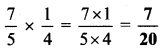ii. $$\frac{6}{7} \times \frac{2}{5}$$iii. $$\frac{5}{9} \times \frac{4}{9}$$iv. $$\frac{4}{11} \times \frac{2}{7}$$v. $$\frac{1}{5} \times \frac{7}{2}$$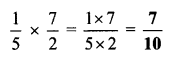vi. $$\frac{9}{7} \times \frac{7}{8}$$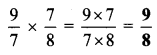vii. $$\frac{5}{6} \times \frac{6}{5}$$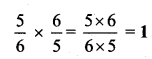viii. $$\frac{6}{17} \times \frac{3}{2}$$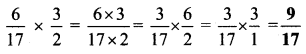Question 2.
Ashokrao planted bananas on $$\frac { 2 }{ 7 }$$ of his field of 21 acres. What is the area of the banana plantation?
Solution:
Area of banana plantation is $$\frac { 2 }{ 7 }$$ of 21
∴ Area of banana plantation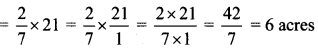∴ Area of banana plantation is 6 acres

Question 3.
Of the total number of soldiers in our army, $$\frac { 4 }{ 9 }$$ are posted on the northern border and one-third of them on the north-eastern border. If the number of soldiers in the north is 5,40,000, how many are posted in the north-east?
Solution:
Number of soldiers posted on northern border = 5,40,000
Since, number of soldiers in north-east = one third of the soldiers on northern border
∴ Number of soldiers in the north-east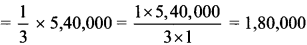∴ The number of soldiers in the north-east is 1,80,000.